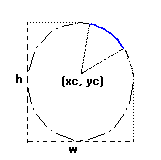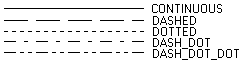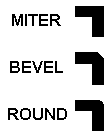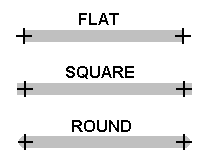## Lines

Line are segments that connects 2 or more points. The Line function includes the 2 given points and draws the line using the foreground color. Line thickness is controlled by the LineWidth function. By using function LineStyle you can draw dashed lines with some variations. Lines with a style other than continuous are affected by the back opacity attribute and by the background color.

```void cdCanvasLine(cdCanvas* canvas, int x1, int y1, int x2, int y2); [in C]
void cdfCanvasLine(cdCanvas* canvas, double x1, double y1, double x2, double y2); [in C]void wdCanvasLine(cdCanvas* canvas, double x1, double y1, double x2, double y2); (WC) [in C]

canvas:Line(x1, y1, x2, y2: number) [in Lua]
canvas:fLine(x1, y1, x2, y2: number) [in Lua]
canvas:wLine(x1, y1, x2, y2: number) (WC) [in Lua]```

Draws a line from (x1,y1) to (x2,y2) using the current foreground color and line width and style. Both points are included in the line.

`Polygons and Bezier Lines`

Open polygons can be created using cdBegin(CD_OPEN_LINES)/cdVertex(x,y)/.../cdEnd().

Closed polygons use the same number of vertices but the last point is automatically connected to the first point. Closed polygons can be created using cdBegin(CD_CLOSED_LINES)/cdVertex(x,y)/.../cdEnd().

Bezier lines can be created using cdBegin(CD_BEZIER)/cdVertex(x,y)/.../cdEnd(). At least 4 vertices must be defined. The two vertices of the middle are the control vertices. A sequence of bezier lines can be defined using more 3 vertices, two control points and an end point, the last point of the previous bezier will be used as the start point.

See the documentation of cdBegin/cdVertex/cdEnd.

```void cdCanvasRect(cdCanvas* canvas, int xmin, int xmax, int ymin, int ymax); [in C]void cdfCanvasRect(cdCanvas* canvas, double xmin, double xmax, double ymin, double ymax); [in C]
void wdCanvasRect(cdCanvas* canvas, double xmin, double xmax, double ymin, double ymax); (WC) [in C]

canvas:Rect(xmin, xmax, ymin, ymax: number) [in Lua]
canvas:fRect(xmin, xmax, ymin, ymax: number) [in Lua]canvas:wRect(xmin, xmax, ymin, ymax: number) (WC) [in Lua]```

Draws a rectangle with no filling. All points in the limits of interval x_min<=x<=x_max, y_min<=y<=y_max will be painted. It is affected by line attributes and the foreground color. If the active driver does not include this primitive, it will be simulated using the cdLine primitive.

```void cdCanvasArc(cdCanvas* canvas, int xc, int yc, int w, int h, double angle1, double angle2); [in C]void cdfCanvasArc(cdCanvas* canvas, double xc, double yc, double w, double h, double angle1, double angle2); [in C]
void wdCanvasArc(cdCanvas* canvas, double xc, double yc, double w, double h, double angle1, double angle2); (WC) [in C]

canvas:Arc(xc, yc, w, h, angle1, angle2: number) [in Lua]
canvas:fArc(xc, yc, w, h, angle1, angle2: number) [in Lua]
canvas:wArc(xc, yc, w, h, angle1, angle2: number) (WC) [in Lua]```

Draws the arc of an ellipse aligned with the axis, using the current foreground color and line width and style.

The coordinate (xc,yc) defines the center of the ellipse. Dimensions w and h define the elliptic axes X and Y, respectively.

Angles angle1 and angle2 are in degrees and oriented counter-clockwise. They define the arc start and end, but they are not the angle relative to the center, except when w==h and the ellipse is reduced to a circle. The arc starts at the point (xc+(w/2)*cos(angle1), yc+(h/2)*sin(angle1)) and ends at (xc+(w/2)*cos(angle2), yc+(h/2)*sin(angle2)). A complete ellipse can be drawn using 0 and 360 as the angles. If angle2 is less than angle1 it will be increased by 360 until it is greater than angle1.

The angles are specified so if the size of the ellipse (w x h) is changed, its shape is preserved. So the angles relative to the center are dependent from the ellipse size. The actual angle can be obtained using rangle = atan2((h/2)*sin(angle), (w/2)*cos(angle)).

To specify the angle in radians, you can use the definition CD_RAD2DEG to multiply the value in radians before passing the angle to CD.

Arc Parameters### Attributes

```int cdCanvasLineStyle(cdCanvas* canvas, int style); [in C]

canvas:LineStyle(style: number) -> (old_style: number) [in Lua]```

Configures the current line style for: CD_CONTINUOUS, CD_DASHED, CD_DOTTED, CD_DASH_DOT, CD_DASH_DOT_DOT, or CD_CUSTOM. Returns the previous value. Default value: CD_CONTINUOUS. Value CD_QUERY simply returns the current value. When CD_CUSTOM is used the cdLineStyleDahes function must be called before to initialize the custom dashes. The spaces are drawn with the background color, except when back opacity is transparent then the background is left unchanged. See BackOpacity.

Line Styles```void cdCanvasLineStyleDashes(cdCanvas* canvas, const int* dashes, int count); [in C]

canvas:LineStyleDashes(dashes: table, count: number) -> (old_style: number) [in Lua]```

Defines the custom line style dashes. The first value is the length of the first dash, the second value is the length of the first space, and so on. For example: "10 2 5 2" means dash size 10, space size 2, dash size 5, space size 2, and repeats the pattern. Sizes are equivalent to pixels, but it may depend on the driver.

```int cdCanvasLineWidth(cdCanvas* canvas, int width); [in C]double wdCanvasLineWidth(cdCanvas* canvas, double width_mm); (WC) [in C]

canvas:LineWidth(width: number) -> (old_width: number) [in Lua]
canvas:wLineWidth(width_mm: number) -> (old_width_mm: number) (WC) [in Lua]```

Configures the width of the current line (in pixels). Returns the previous value. Default value: 1. Value CD_QUERY simply returns the current value. Valid width interval: >= 1.

In WC, it configures the current line width in millimeters.

```int cdCanvasLineJoin(cdCanvas* canvas, int style); [in C]

canvas:LineJoin(style: number) -> (old_style: number) [in Lua]```

Configures the current line style for: CD_MITER, CD_BEVEL or CD_ROUND. Returns the previous value. Default value: CD_MITER. Value CD_QUERY simply returns the current value.

Line Joins```int cdCanvasLineCap(cdCanvas* canvas, int style); [in C]

canvas:LineCap(style: number) -> (old_style: number) [in Lua]```

Configures the current line style for: CD_CAPFLAT, CD_CAPSQUARE or CD_CAPROUND. Returns the previous value. Default value: CD_CAPFLAT. Value CD_QUERY simply returns the current value.

Line Caps